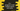# C++ program to read student names and marks using structure## Introduction:

In this C++ tutorial, we will learn how to read the names and marks of a list of students by using a `structure`. We will create one `structure` that can be used to hold the name and marks for one student and we will create one `array` of that structure to hold the names and marks of a list of students.

This program will take all inputs from the user. We are explaining everything at the bottom of this post. You can try to run this program with different inputs.

### C++ program to read the names and marks of a list of students:

``````#include <iostream>
using namespace std;

// 1
struct student
{
string name;
int totalSubjects;
int marks;
};

// 2
void printStudentDetails(student students[], int totalStudents)
{
// 3
for (int i = 0; i < totalStudents; i++)
{
cout << "Student name: " << students[i].name << endl;
cout << "Marks:" << endl;
for (int j = 0; j < students[i].totalSubjects; j++)
{
cout << students[i].marks[j] << endl;
}
}
}
int main()
{
// 4
int totalStudents;

cout << "Enter total number of students: ";

cin >> totalStudents;

// 5
if (totalStudents <= 0)
{
cout << "Please enter a valid number" << endl;
return -1;
}

student studentArray[totalStudents];

// 6
for (int i = 0; i < totalStudents; i++)
{
// 7
cout << "Enter the name of student " << (i + 1) << ":" << endl;
cin >> studentArray[i].name;

cout << "Enter total number of subjects: ";
cin >> studentArray[i].totalSubjects;

// 8
if (studentArray[i].totalSubjects > 20 || studentArray[i].totalSubjects <= 0)
{
cout << "Please enter a valid number" << endl;
return -1;
}

// 9
for (int j = 0; j < studentArray[i].totalSubjects; j++)
{
cout << "Enter marks for subject " << (j + 1) << ":" << endl;
cin >> studentArray[i].marks[j];
}
}
// 10
printStudentDetails(studentArray, totalStudents);
}``````

#### Explanation :

The commented numbers in the above program denote the step numbers below:

1. The `student` is a structure. We will create one array of this structure type to hold the information of all students. It has three members: the `string` member `name` is used for the name of the student, the `int` member `totalSubjects` is used for the total number of subjects for the student, and the `int` array `marks` is used for the marks of each subject.
2. The `printStudentDetails` function prints the details of a `student` array. It has two parameters: the array of students and the total number of students. It loops through the array of students and prints the contents.
3. We are using one `for` loop to iterate through the student array. This loop runs from `i = 0` to `i = totalStudents - 1`. Inside the loop, we are printing down the name of the student and the marks of the subjects. One more inner `for` loop is used to iterate through all subjects and it will print all marks. For each iteration of the outer loop, the inner loop runs for `totalSubjects` number of times for that student.
4. Inside the main function, we have one integer variable `totalStudents` to assign the total number of students. This value is entered by the user.
5. This is a safety check. If the user enters an invalid number for total students, it will quit the program. If the entered number is valid, create one array to hold the information of the students.
6. Start one `for` loop to iterate through the array of students one by one.
7. Inside the loop, ask the user to enter the name of the student. Read and assign it to the `name` member for that specific array element. Similarly, read the total number of subjects.
8. This is another safety check. The total subjects should be greater than 0 and less than 20 because our `marks` array in the structure can hold a maximum of 20 elements.
9. Use one `for` loop to read the marks of each student. This is an inner loop. For each student, it will run for `totalSubjects` number of times. Get the marks from the user one by one.
10. Finally, call the `printStudentDetails` function to print the details.

#### Sample Output :

``````Enter total number of students : 2
Enter the name of student 1 :
Alex
Enter total number of subjects : 2
Enter marks for subject 1 :
40
Enter marks for subject 2 :
45
Enter the name of student 2 :
Bob
Enter total number of subjects : 3
Enter marks for subject 1 :
50
Enter marks for subject 2 :
54
Enter marks for subject 3 :
56
Student name : Alex
Marks :
40
45
Student name : Bob
Marks :
50
54
56``````

### Conclusion :

You can add an unlimited number of students and up to 20 subject marks. You can download the program on GitHub and please raise a PR if you have any better solution.